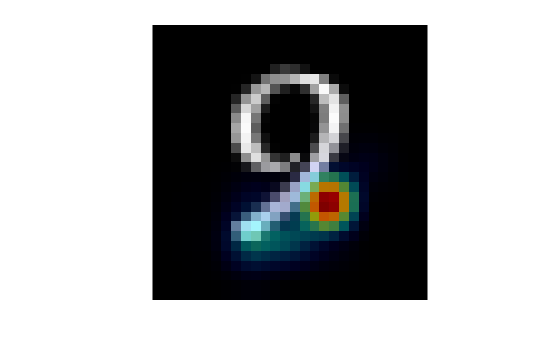# anomalyMapOverlay

Overlay heatmap on image using per-pixel anomaly scores

Since R2022b

## Syntax

``B = anomalyMapOverlay(I,map)``
``B = anomalyMapOverlay(I,map,Name=Value)``

## Description

example

````B = anomalyMapOverlay(I,map)` fuses an overlay of the per-pixel anomaly scores in `map` as a heatmap on a grayscale or RGB background image, `I`. NoteThis functionality requires the Computer Vision Toolbox™ Automated Visual Inspection Library. You can install the Computer Vision Toolbox Automated Visual Inspection Library from Add-On Explorer. For more information about installing add-ons, see Get and Manage Add-Ons. ```
````B = anomalyMapOverlay(I,map,Name=Value)` specifies options using one or more name-value arguments. For example, `anomalyMapOverlay(I,map,Blend="equal")` blends the score map and the background image with equal weighting.```

## Examples

collapse all

Load a pretrained FCDD anomaly detector. This detector has been trained to classify the digit 8 as normal and all other digits as anomalies.

`load("digit8AnomalyDetector.mat");`

Load test images and corresponding labels, then convert the test images to a formatted `dlarray` with the format label "SSCB" for spatial-spatial-channel-batch. The data set consists of grayscale images of handwritten digits 0–9.

`[Xtest,gtLabels] = digitTest4DArrayData;`

Select a test image of the digit 9.

```digitToTest = "9"; digitIdx = find(gtLabels==digitToTest,1); imTest = Xtest(:,:,:,digitIdx);```

Predict the per-pixel anomaly scores for the test image.

`map = anomalyMap(detector,imTest);`

Display the anomaly map over the test image.

```overlay = anomalyMapOverlay(imTest,map); imagesc(overlay) axis image off```## Input Arguments

collapse all

Background image, specified in one of these formats:

• M-by-N-by-3 numeric array representing a truecolor image

• M-by-N numeric matrix representing a grayscale image

• M-by-N logical matrix representing a binary image

Anomaly score map, specified as an M-by-N numeric matrix. You can get an anomaly score map using the `anomalyMap` function. Larger values indicate a greater chance that a pixel is an anomaly.

### Name-Value Arguments

Specify optional pairs of arguments as `Name1=Value1,...,NameN=ValueN`, where `Name` is the argument name and `Value` is the corresponding value. Name-value arguments must appear after other arguments, but the order of the pairs does not matter.

Example: `B = anomalyMapOverlay(I,map,Blend="equal")` blends the score map and the background image with equal weighting.

Display range of the anomaly score map, `map`. The default value is `[min(map(:)) max(map(:))]`.

Weights to blend the map with background image `I`, specified as `"proportional"`, `"equal"`, or a numeric scalar in the range [0, 1].

Value Description
`"proportional"`Overlay `I` and `map` using alpha blending with per-pixel weights proportional to the corresponding values in `map`. The heatmap is more opaque over regions of interest, which have large anomaly scores. The heatmap is more transparent over normal regions, which have small anomaly scores.
`"equal"`Overlay `I` and `map` using alpha blending with equal weights for the map and background image.
numeric scalarOverlay `I` and `map` using alpha blending with weight w for the map, where w is the value of the `Blend` argument. When w is `0`, the heatmap is fully transparent and only the background image is visible. When you increase the value of w, the heatmap becomes more opaque.

For all values of `Blend`, the weight of the heatmap has an additional multiplicative scale factor, specified by `BlendFactor`. When you account for the additional scale factor, the weight of the heatmap is w*`BlendFactor` and the weight of the background image is 1 – w*`BlendFactor`.

Constant multiplicative factor to apply to the weights of the `Blend` argument, specified as a numeric scalar. Increasing the value of `BlendFactor` increases the brightness of the heatmap over the image.

Example: `BlendFactor=1.2` brightens the heatmap by increasing the weighting by a factor of `1.2`.

Example: `BlendFactor=0.6` darkens the heatmap by decreasing the weighting by a factor of `0.6`.

Colormap, specified as a 256-by-3 numeric matrix with values in the range [0, 1]. Each row is a three-element RGB triplet that specifies the red, green, and blue components of a single color of the colormap.

Data Types: `single` | `double`

## Output Arguments

collapse all

RGB image with heatmap overlay, returned as an M-by-N-by-3 numeric array.

Data Types: `uint8`

## Version History

Introduced in R2022b